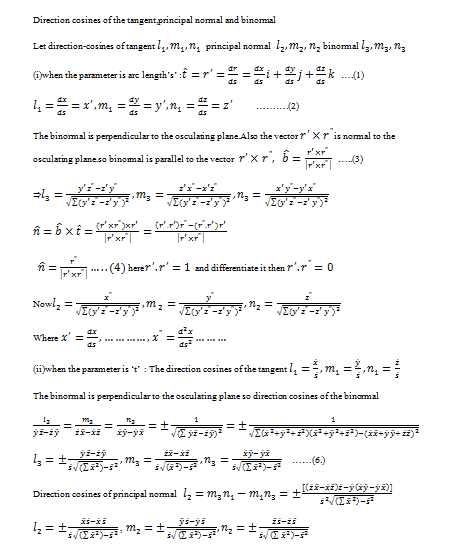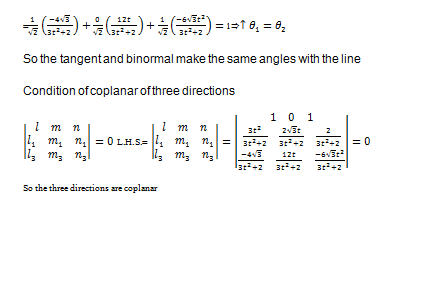z35W7z4v9z8w Direction cosines of the tangent principal normal and binormal

Direction cosines of the tangent principal normal and binormal

(2.)Binormal:The normal perpendicular to the principal normal of a given curve at a point p is called binormal of the curve at that point.Direction cosines of the tangent,principal normal and binormal

(3.)Angles between tangent and the given line,Angles between binormal and the given line(4.)The condition of the three directions are coplanar